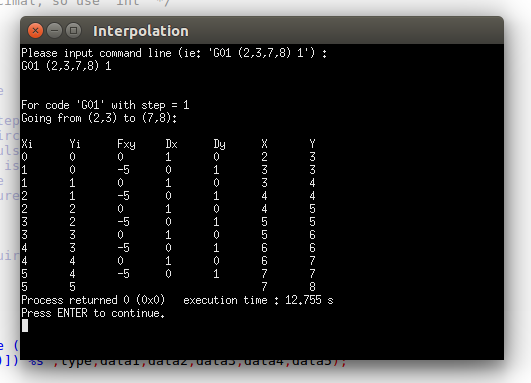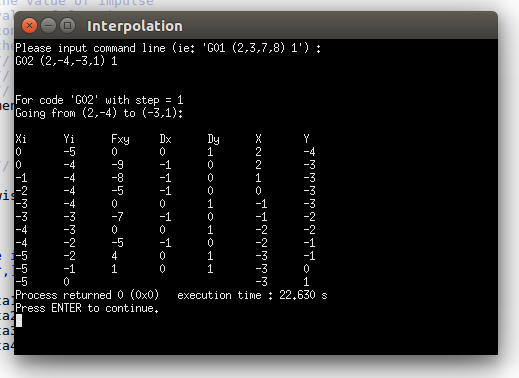# Line and Circular Interpolation on XY Plane

Tips: This is my homework of “Computer Control System” course, so mistakes do happen.

This article will discuss an interpolation algorithms for line and circular. And it provides an implement of C language.

### Interpolation Algorithms

Many numerical control machines are powered by stepping motors. When a pulse is sent to a stepping motor, the stepping motor alters its position by a unit step. Two motors can be used to control the XY movements of an arm or tool over a working plane.

If the pulse are generated by a device which can remember or generate a specified train of pulses, repetitive operations such as grinding, painting, or cutting can be performed hunderds of times with virtually no variation. A microcomputer is an obvious choice to gernerate and remember the pulse.

Since stepper motors can move only in discrete steps, we must approximate the actual curve by a series of small XY motions. Many algorithms rely upon parametric functions such as sine and cosine to perform the necessary calculations. Parametric functions, however, typically require a high degree of numeric precision. Calculating sine and cosine values with a microcomputer can be too time-consuming to be useful in a realtime application.

The following two algorithms require no parametric functions. This makes them ideally suited to the
computation and memory capacities of microcomputers. Since these algorithms do not require a large amount of complex mathematical calculation, they are fast enough to be used in real-time applications.

### Result### Reference

“XY INTERPOLATION ALGORITHMS” By Kenneth and Melvin Goldberg